Thread: Eric Dollard View Single Postt-rexSenior Member Join Date: Aug 2011 Location: In the bushes Posts: 422
The Law of E.M. Induction, Part 6 (1 of 3)

(1) Steinmetz closes chapter three in his A.C. book, 1916 edition, with his development of the concept of Inductance. Here given, for the first time in chapter three, is the current, i, in ampere. It is the electronic current, for the lack of a better term. Steinmetz gives the relation,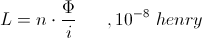This is the Magnetic Inductance, also known as the co-efficient of magnetic energy storage.

Steinmetz complicates this relation by giving the current, i, in effective values, an unnatural form. The relation is given by,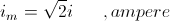Hence the resulting expression is given by,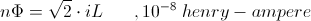Transposing his relation gives,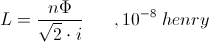This derives from Steinmetz's expression the co-efficient of self induction.

Using maximum rather than effective values for current simplifies the relation to,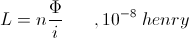This the basic expression for the co-efficient of self induction.

(2) This self induction is a non motional, or static, induction. This represents the reactance coil. It is important to notice that the self induction is distinct from the motional induction produced by the dynamo. The motional relation, that of rotary motion, is given by the relation,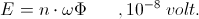The induction for the static, or stationary, condition is given by the relation,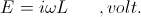In the static expression the number of turns, n, and the factor of ten to the minus eighth, are absorbed into the henry, that is,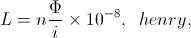becomes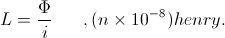(3) Continuing in chapter three Steinmetz introduces the concept of Reactance. The relation is given by,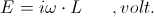Substituting the relation,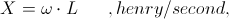Establishes the basic Ohm's Law expression for alternating currents,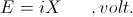X is called the reactance of the coiled winding. Transposing gives the expression for the reactance as,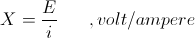And it is that volt per ampere defines the dimensions of the ohm. This volt per ampere can also be expressed as a henry per second, giving the relations,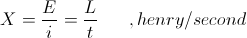And it is,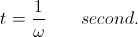This expression of reactance can be considered a synchronous inductance, this in an A.C. circuit.
__________________
SUPPORT ERIC DOLLARD'S WORK AT EPD LABORATORIES, INC.

Purchase Eric Dollard's Books & Videos - 70% of the sale goes to Eric and EPD Laboratories: Eric Dollard Books & Videos
Donate by Paypal: Donate to EPD Laboratories